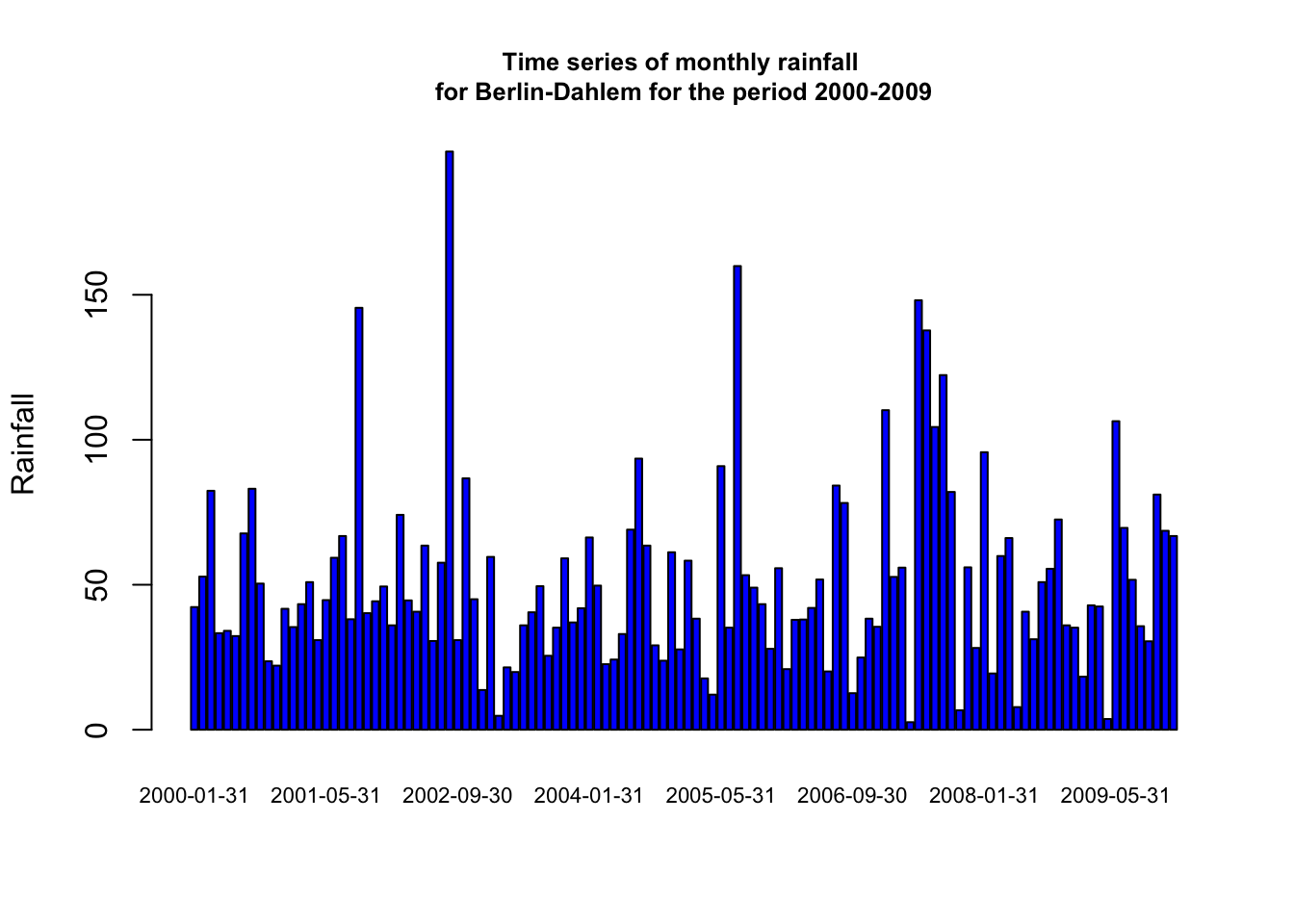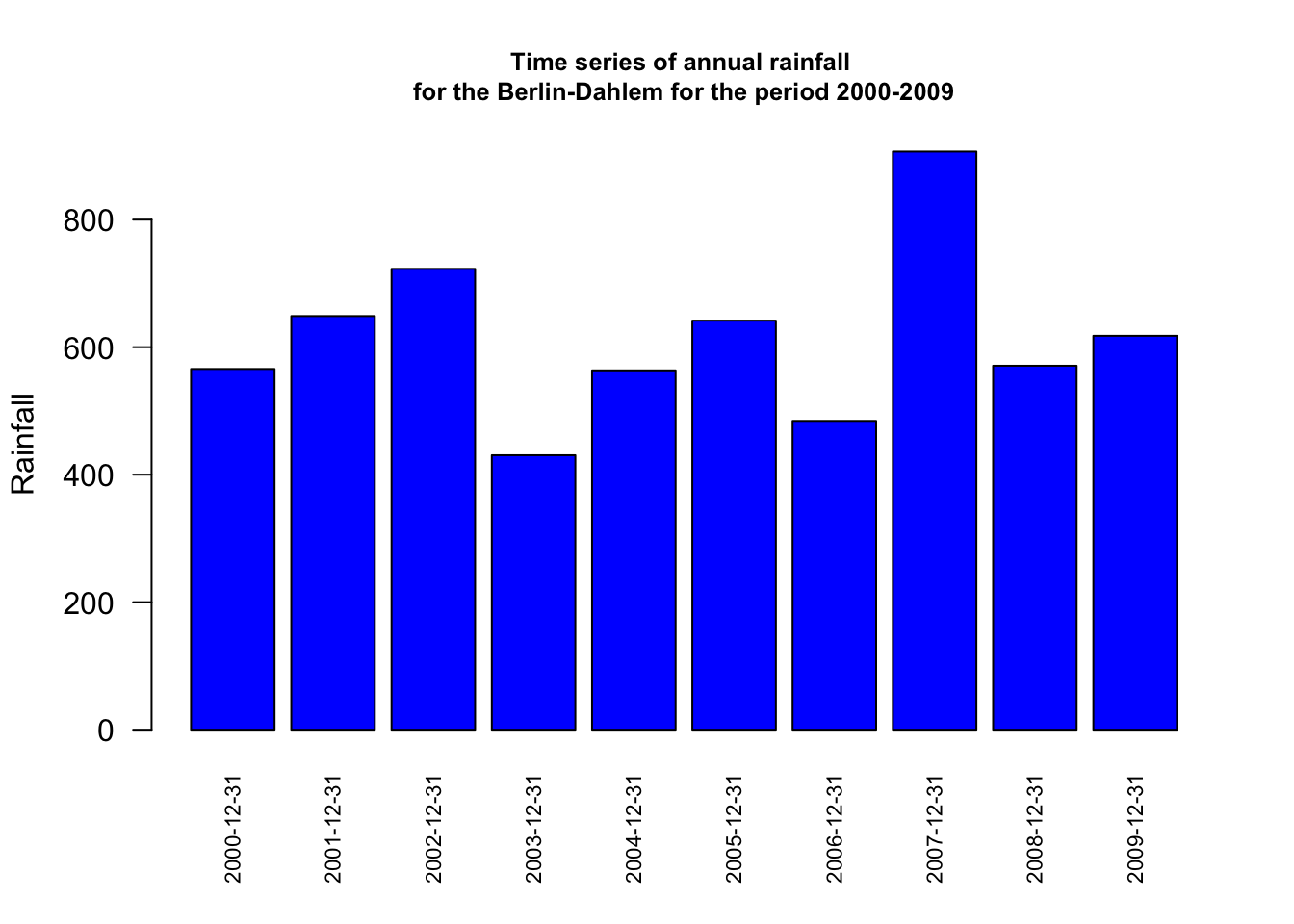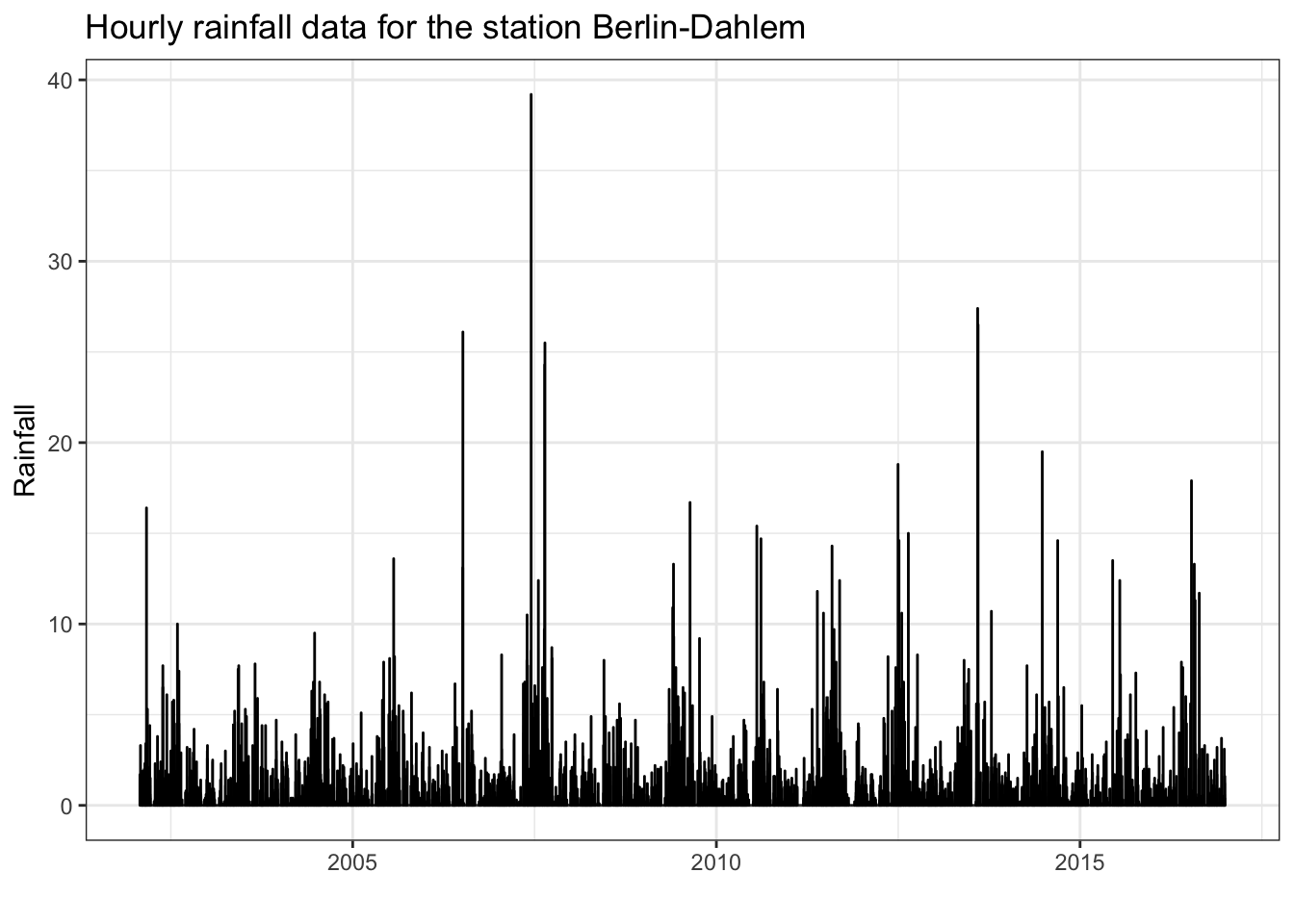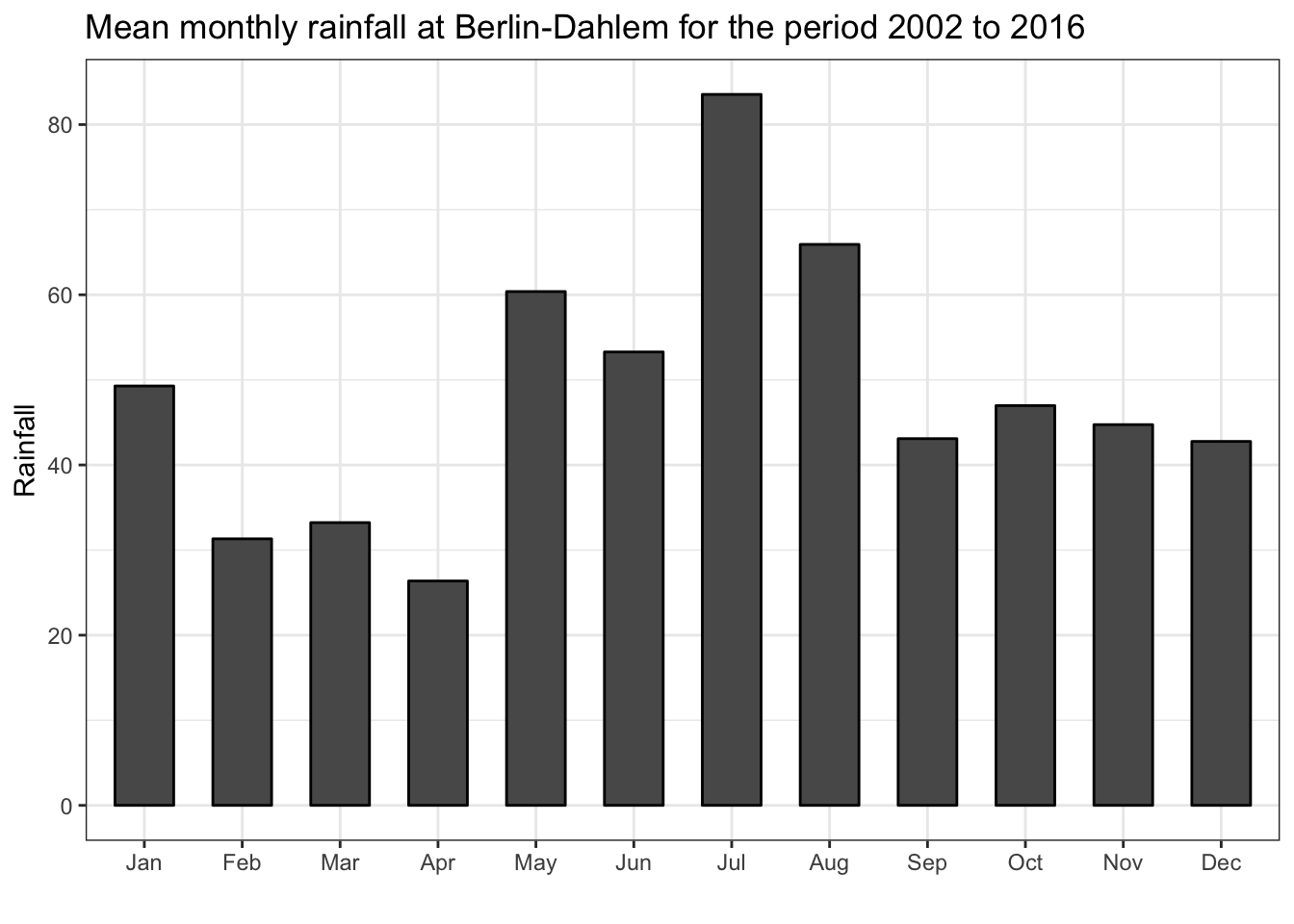Sometimes it is necessary to reshape the time series to coarser intervals than given. Another very useful bundle of functions for the aggregation of time series data is provided by the `xts` package. These functions are:

• `apply.daily(ts, func)`
• `apply.weekly(ts, func)`
• `apply.monthly(ts, func)`
• `apply.quarterly(ts, func)`
• `apply.yearly(ts, func)`

This family of functions applies a function to each distinct period in a given time series object. Thus, we may easily calculate a time series of monthly or annual rainfall based on daily data:

``````library(xts)
library(lubridate)
## subset the data
daily.2000.2009 <- ts.FUB.daily['2000/2009', ]

### monthly time series ###
mothly.rain <- apply.monthly(daily.2000.2009[, 'Rain'], sum)

barplot(mothly.rain,
main = "Time series of monthly rainfall \nfor Berlin-Dahlem for the period 2000-2009",
col = 'blue',
cex.main = 0.8, # font size title
ylab = 'Rainfall',
cex.names = 0.7) # font size x-labels````````````### annual time series ###
annual.rain <- apply.yearly(daily.2000.2009[, 'Rain'], sum)

barplot(annual.rain,
main = "Time series of annual rainfall \nfor the Berlin-Dahlem for the period 2000-2009",
col = 'blue',
cex.main = 0.8,
ylab = 'Rainfall',
cex.names = 0.7,
las = 2) # rotate label x-axis``````#### Split-Apply-Combine

So far we learned about summarizing and aggregating time series data. Now we combine these approaches in order to get a more sophisticated representation of our data. Therefore we incorporate basic ideas of the so called split-apply-combine strategy for data wrangling.

We elaborate this analytic strategy in two exercises using hourly rainfall data for the station Berlin-Dahlem.

``````library(ggfortify)
autoplot(ts.FUB.hourly) +
labs(y = 'Rainfall') +
ggtitle('Hourly rainfall data for the station Berlin-Dahlem') +
theme_bw()``````1. Exercise

Our goal is calculate the mean monthly rainfall at the weather station Berlin-Dahlem for the period 2002 to 2016.

Our analytic strategy can be summarized as

• split data into pieces (into months),
• apply some function to each piece (sum up the amount of rainfall),
• combine the results back together again (plot the data or store the data for further usage).

For sure we could achieve that task by writing `for` loops, but we will probably spend a lot of time on bookkeeping tasks. In base R there exists the family of `apply` functions, such as `apply()`, `aggregate()`, `tapply()`, and `by()`, among others. These are, no doubt, excellent tools, however, thanks to Hadley Wickham the author of “The split-apply-combine strategy for data analysis”, and software developer of major data analysis tools for R, we may use a collection of R packages, sometimes referred to as tidyverse packages. Out of those we pick the `dplyr` package, a package for data manipulation. You can learn more about the `dplyr` package by typing `vignette("dplyr")` into your console.

In addition to the functionality of the `dplyr` package we make use of the pipe operator (`%>%`). This allows us to write less code which is even more readable. Learn more on the pipe operator by visiting the free online book R for Data Science by Garrett Grolemund and Hadley Wickham (Chapter 18: Pipes). First we load the required packages.

``library(dplyr)``

Then we stack the time series data, which is of object class `xts`, into a data frame, as the `dplyr` package is optimized to work on `data.frame` objects.

``````# stack time series data into a data frame
df.hour <- data.frame('Datetime' = index(ts.FUB.hourly),
coredata(ts.FUB.hourly))``````

Now we run the analysis:

``````# initial dataframe
mean.monthly.rainfall <- df.hour %>%
#### DATA WRANGLING ####
# generate two more columns and populate them with the year and month
mutate(year = year(Datetime),
month = month(Datetime)) %>%
# group the data by these new columns
group_by(year, month) %>%
# calculate the monthly rainfall for each year
summarise(year.month.rainfall = sum(Rain))  %>%
# group the data by the month
group_by(month) %>%
# calculate the mean annual monthly rainfall
summarise(months.count = n(),
mean.month.rainfall = mean(year.month.rainfall, na.rm = T))
mean.monthly.rainfall``````
``````## # A tibble: 12 x 3
##    month months.count mean.month.rainfall
##    <dbl>        <int>               <dbl>
##  1     1           15                49.3
##  2     2           15                31.3
##  3     3           15                33.2
##  4     4           15                26.4
##  5     5           15                60.4
##  6     6           15                53.3
##  7     7           15                83.5
##  8     8           15                65.9
##  9     9           15                43.1
## 10    10           15                47.0
## 11    11           15                44.7
## 12    12           15                42.8``````

We wrote in total five lines of code and calculated the mean monthly rainfall at the weather station Berlin-Dahlem for the period of 15 years based on hourly data.

Now we visualize the data using the `ggplot()` function.

``````mean.monthly.rainfall %>%
#### PLOTTING ####
# add column with month names for labeling
mutate(label.month = month(month,label = TRUE, abbr = TRUE)) %>%
# plot using ggplot()
ggplot(aes(x = label.month, y = mean.month.rainfall)) +
# design the bar plot
geom_bar(stat = "identity", width = 0.6, color = "black") +
# label axes
labs(y = "Rainfall", x = "") +
ggtitle(paste('Mean monthly rainfall at Berlin-Dahlem for the period',
year(xts::first(ts.FUB.hourly)), # calculate start year of series
'to',
year(xts::last(ts.FUB.hourly)))) + # calculate end year of series
# pick black and white background theme
theme_bw()``````2. Exercise

Our goal of the second exercise is to calculate the mean diurnal monthly rainfall at Berlin-Dahlem for the period 2002 to 2016.

Again we make use of the `dplyr` package and the pipe operator `%>%`. The code is very similar to the code from above, however with minor modifications we can solve a very distinct data analysis task.

``````df.hour %>%
### DATA WRANGLING ###
mutate(month = month(Datetime),
hour = hour(Datetime)) %>%
group_by(month, hour) %>%
summarize(sum.hour = sum(Rain, na.rm = TRUE)) %>%
mutate(sum.hour = sum.hour/15) %>% # normalize to annual mean
### PLOTTING ###
mutate(label.month = month(month, label = TRUE, abbr = TRUE)) %>%
ggplot(aes(x = hour, y = sum.hour)) +
geom_bar(stat = "identity", width = 0.1, color = "black") +
facet_wrap( ~ label.month, ncol = 4) +
labs(y = "Rainfall (mm)", x = "Hour of the day") +
ggtitle(paste('Mean diurnal monthly rainfall at Berlin-Dahlem for the period',
year(xts::first(ts.FUB.hourly)),
'to',
year(xts::last(ts.FUB.hourly)))) +
theme_bw()``````